• 1. 用C/C++语言编写方法的化简和改造程序，实现以下功能之一（如实现两个功能，则满分为110分；如实现三个功能，则满分为120分）： (1) 无用符号和无用产生式的删除，参考课本中算法2.1和算法2.2。 (2) ε-产生式的...
• 文法化简与改造1、无用符号及无用产生式的删除 无用符号：设有一文法G[S]= （VN ，VT ，P，S），说G中的一个符号X∈V是有用的是指X至少出现在一个句子的推导过程中，即满足：存在α，β∈V*，有S=*>αXβ 存在ω∈...
文法的化简与改造

1、无用符号及无用产生式的删除
无用符号：设有一文法G[S]= （VN ，VT ，P，S），说G中的一个符号X∈V是有用的是指X至少出现在一个句子的推导过程中，即满足：
存在α，β∈V*，有S=*>αXβ
存在ω∈VT* ，αXβ=*>ω
否则X为无用符号

设有文法G[S]= （VN ，VT ，P，S），首先用算法2.1改造该文法的到G1[S]= （VN1 ，VT ，P1，S），使得对于每一个X∈VN1，都有ω∈VT*，X=*>ω

算法1：
分别置VN1，P1为Φ。
对P中每一个产生式A→δ，若δ∈VT*，则将A放入VN1中。
对P中每一个产生式A→X1 X2……XK，若每一个Xi 都属于VT或VN1，则将A放入VN1中。
重复③直至VN1不增大。
对于P中的每一个产生式B→Y1 Y2……Yn ，若B及每一个Yi ，都属于VN1∪VT  ，则将B→Y1 Y2……Yn，放入P1中。
其次，对以给文法G[S]，若执行算法2.2可得到一等价文法G’=（VN’， VT’ ，P’，S）使得对任一X∈VN’∪ VT’都存在α，β∈（VN’∪ VT’）有S=*>αXβ.
1.分别置VN’、 VT’、P’为φ

算法2:

2.将S 放入VN’中。
3.对于G中任何型如A→α1|……|αm的产生式，若A∈VN’则将α1……αm 中的全部非终结符放入VN’中，终结符放入VT’中。
4.重复③直至VN’、 VT’不增大为止。
5.将P中左右部仅含VN’∪ VT’中符号的所有产生式放入P’ 中。

2、ε—产生式的消除

有的分析方法要求文法中不能含有ε—产生式，因此需要改造文法使之不含ε—产生式。
如果语言不含有ε句子，则可有办法消除文法中的全部ε—产生式，否则不可能全部消除，但我们希望只有在空句子的推导中用到ε—产生式，其他语句的推导过程中不会使用ε—产生式。故对含有空句子的文法，我们希望只有文法开始符S→ε这样一个产生式并且S不出现在其它任何产生式的右部。

算法3：
找出所有能导出ε的非终结符。
1.构造W1={A|产生式A→ε∈P}
2.构造集合序列WK+1= WK∪{B|B→β∈P，且β∈WK+，K≥1}
WK+1是一个有限集，设最后的WK+1为W。
当S∈W时，ε∈L（G[S]）。

设有一文法G[S]= （VN ，VT ，P，S），当ε不属于该文法所描述的语言时，可构造文法：
G’=（VN，VT，P’，S），使得L（G’）=L（G），G’不含有ε产生式：

算法4:
1.利用W将VN 分为两个子集W及VN -W。
2.设A→X1 X2……XK∈P，按下面规则将所有型如A→Y1 Y2……YK 的产生式放入P’中，对于一切1≤i≤k：
a．若Xi 不属于W，则取Yi = Xi
b．  若Xi ∈W，则分别取Yi 为Xi与ε，但是若所有Xi均属于W，却不能把所有Yi 取为ε。

设有一文法G[S]= （VN ，VT ，P，S），当ε属于该文法所描述的语言时，可构造文法：
G1=（VN1 ，VT ，P1，S’），使得L（G1）=L（G），P1中除S’→ε外不再含有其它ε产生式，并且S’不出现在任何产生式的右边。

算法5：
若S不出现在任何产生式的右部，则可直接用算法2.4消除ε产生式，再加入S→ε，否则：
1．引入新的非终结符S’， VN1= VN ∪{ S’}
2．构造P’ =P∪{ S’→α| S→α∈P}
3．对文法G1=（VN1 ，VT ，P1，S’），执行算法4，再加入S’→ε。

3、单产生式的消除
A→B，A,B∈VN 此类产生式被称为单产生式。
假定文法中不含有ε产生式。

算法6：
设VN ={ A1 ...... AN } 对每一个Ai （1≤i≤n）构造集合序列
W1( Ai）={Ai}，
WK+1（Ai ）= WK（Ai ）∪{D|C→D∈P，C∈WK（Ai ），D∈VN }
K≥1，该集合序列存在一个j，有
Wj（Ai ）= Wj+1（Ai ）......
令W（i）= Wj（Ai ）
W（i）={B| Ai =>B，B∈VN }
构造P’=∪{ Ai →α|B→α∈P，B∈W（i），α不是单个非终结符}

=================================================================
附：源代码，请在此下载
http://d.download.csdn.net/down/309862/njdragonfly
展开全文算法 语言
• 文法和语言的基本知识——基本知识要点汇总，主要对字母表、符号、符号串、文法、语言等相关基本概念进行了汇总，针对文法的形式定义、语言的形式定义、语法分析树、文法的二义性、文法化简等重点基本问题进行详细...

halo~我是bay_Tong桐小白 本文内容是桐小白个人对所学知识进行的总结和分享，知识点会不定期进行编辑更新和完善，了解最近更新内容可参看更新日志，欢迎各位大神留言、指点

文法和语言的基本知识——基本知识要点汇总
【更新日志】

程序设计语言的描述字母表、符号、符号串基本概念运算法则
文法的形式定义语言的形式定义语法分析树文法化简变换文法的二义性文法和语言的分类常见题型

【更新日志】
最近更新：
暂无编辑内容，持续更新中……
程序设计语言的描述
程序设计语言的三个考虑因素：语法、语义、语用
语法：对语言结构的定义语义：描述了语言的含义语用：从使用的角度描述语言
非形式化定义与形式化定义
非形式化定义：自然语言描述形式化定义：用符号式子组成的序列描述
字母表、符号、符号串
基本概念
字母表： 字母表 ∑ 是一个元素的非空有穷集合
非空——至少包含一个元素元素——可以是字母、数字或其他符号
符号（字符）： 字母表中的元素
符号串（字）： 指字母表中符号的有穷序列
建立在指定字母表上，有字母表上的有穷个符号组成符号串中符号的顺序不可忽视，如 ab 与 ba 是字母表上的两个不同的符号串
串的长度： 符号串中符号的长度。eg：串 s 的长度，通常记作|s|
不包含任何符号的符号串称为符号串，其长度为0，用 ε 表示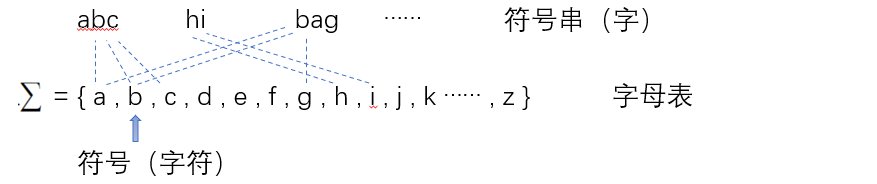运算法则
符号串的连接： 设 x 和 y 是符号串，则串 xy 称为它们的连接。eg：设 x = abc，y = 10a，则 xy = abc10a，yx = 10aabc
空串是连接运算的单位元，即对任意一个符号串x，有 ε x = x ε = x设 x,y,z是三个字符串，如果x=yz，则称y是x的前缀，z是x的后缀
符号串的幂运算： 串s的n次幂，即将n个s连接起来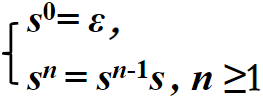集合的乘积：（以字母表运算为例）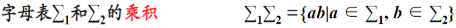集合的幂运算： （以字母表运算为例）字母表 ∑ 的 n 次幂， 即长度为n的符号串构成的集合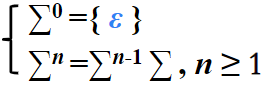集合的正闭包与闭包：（以字母表运算为例）
正闭包：字母表 ∑ 的正闭包，即长度正数的符号串构成的集合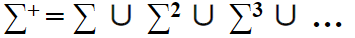闭包（也称克林闭包）：字母表 ∑ 的克林闭包， 即任意符号串构成的集合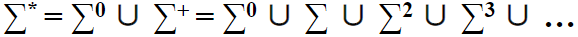注：符号串的集合和元素的集合（即字母表）都遵循此集合运算法则
文法的形式定义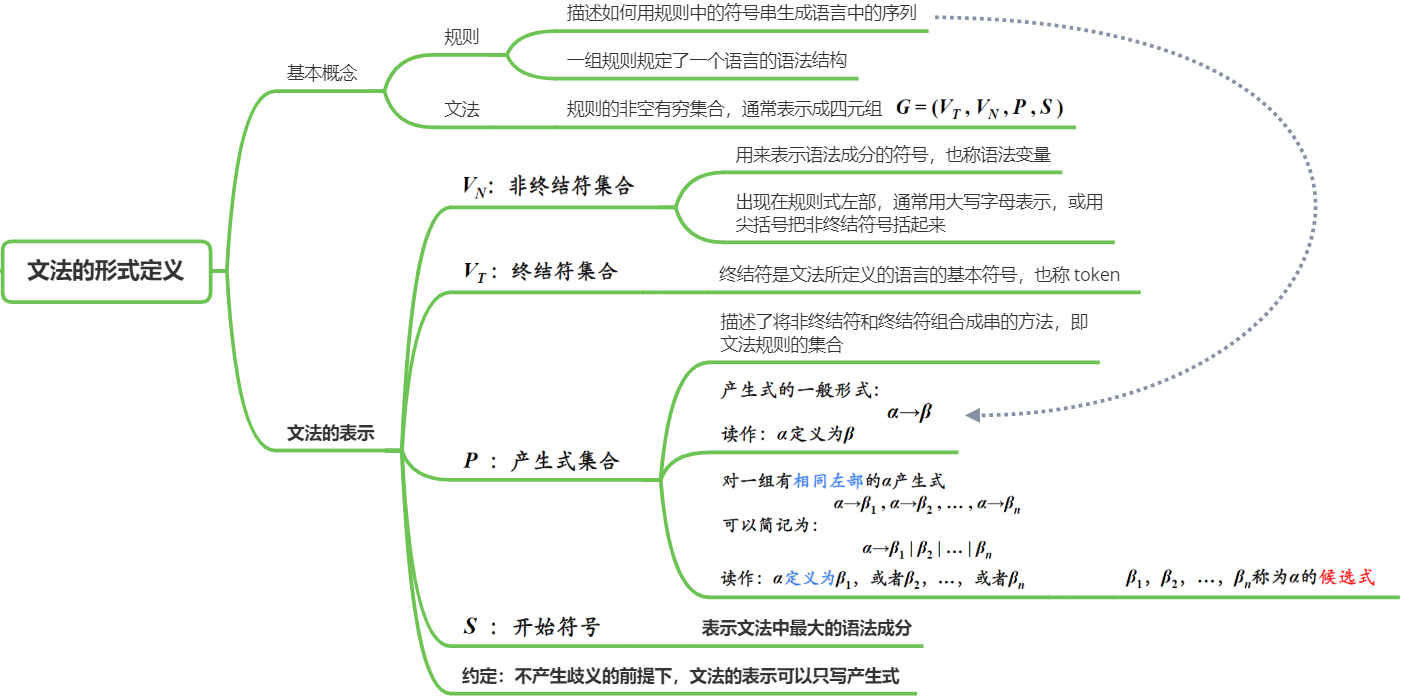语言的形式定义
推导： 从文法开始符号开始，通过产生式的右部取代左部，最终产生句子（由终结符构成的符号串）的过程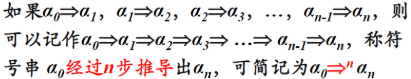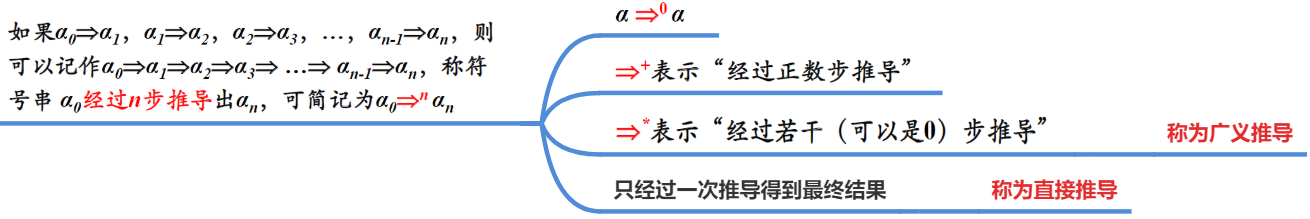规约： 从给定源语言的句子开始，通过产生式的左部取代右部，最终到达开始符号的过程
推导和规约的关系：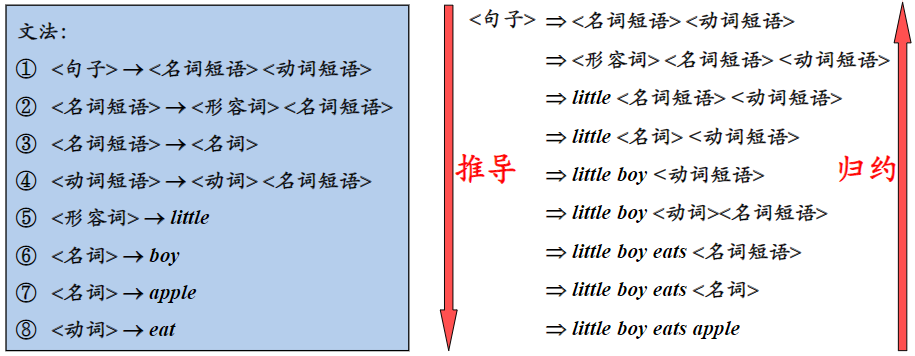推导和规约的每一步只能用一个产生式进行替换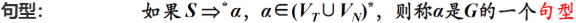即一个句型中既可以包含终结符，又可以包含非终结符，也可能是空串即句子是不包含非终结符的句型
语言： 由文法 G 的开始符号 S 推导出的所有句子构成的集合称为文法 G 生成的语言，记为L(G)
L( G[S] ) = { x|S正数步推导到x 且 x∈终结符号集合闭包}
【ps：S 经过正数步推导得到 x，而不是用广义推导】
∵ S∈非终结符号集合
若用广义推导，则有可能有S=x
则与x属于终结符号集合闭包相矛盾

由文法确定语言的中心思想：从文法符号出发，反复连续地使用规则，对非终结符施行替换和展开，找出句子的规律，用式子或自然语言描述出来给定一个文法，就能从结构上唯一地确定其语言（即当文法给定，语言也就确定）给定一种语言，能确定其文法，但文法不是唯一的若G和G’是两个不同的文法，如果它们描述的语言相同，那么G和G‘为等价文法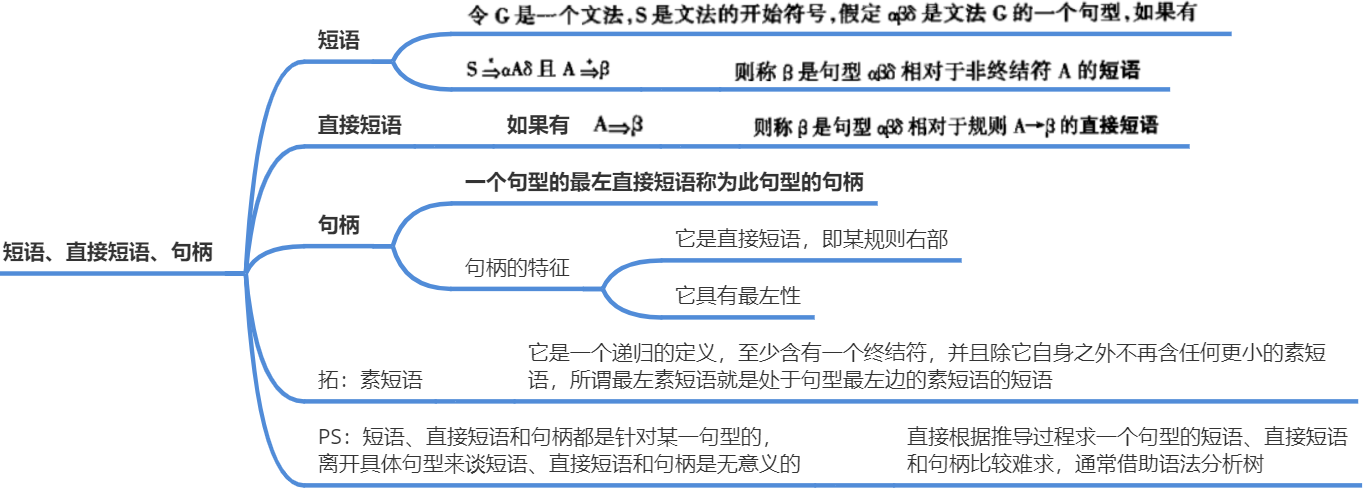最左推导： 对于一个推导序列中的每一步直接推导，都是对最左非终结符进行替换
最右推导： 对于一个推导序列中的每一步直接推导，都是对最右非终结符进行替换
也称规范推导用规范推导推导出的句型称为规范句型规范推导的逆过程称为最左规约，也称为规范规约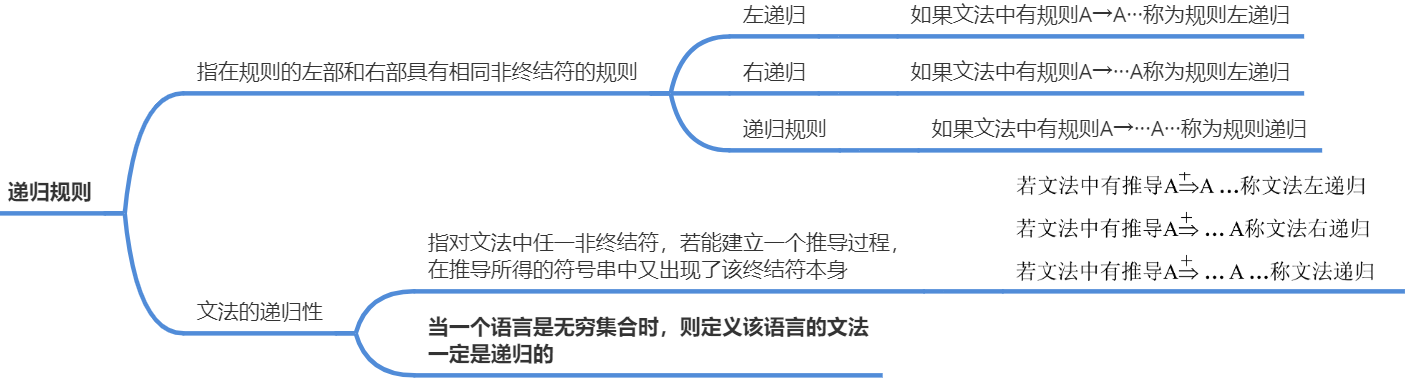语法分析树
语法分析树： 对句型的推导过程给出一种图形表示，这种表示称为语法树，也称推导树；从左到右排列叶节点得到的符号串称为是这棵树的产出或边缘
语法分析树特点：
每个结点的符号均包含在集合V中文法的开始符号作为树根若某一结点至少有一个分支节点，则该节点上的标记一定是非终结符若A的结点有k个分支结点，则 A→A1A2···Ak 一定是G的一条规则若该语法树中的叶子节点自左向右排列为w，则w为该文法的句型；若w中所有符号都是终结符，则w是该文法的一个句子
子树： 由某一结点连同其所有分支组成的部分
简单子树： 只有单层分支的子树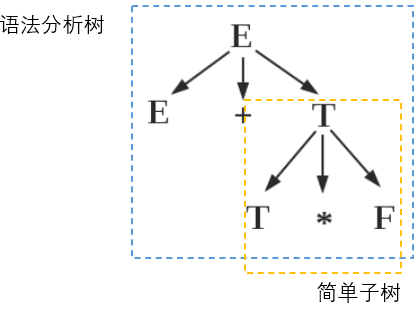短语、直接短语、句柄的直观解释：
短语：子树的末端结点形成的符号串是相对于子树根的短语直接短语：简单子树的末端结点形成的符号串是相对于简单子树根的直接短语句柄：最左简单子树的末端结点形成的符号串是句柄
文法化简变换
文法中不能含有形如 A→A 的规则，这种规则称为有害规则，没有必要存在，且还会引起文法的二义性文法中不能有在推导文法的所有句子中始终都不可能用到的规则对文法中的某个非终结符A无法从它推导出任何终结符号串来，这样的规则不能出现。如 C→Ce
文法的二义性
文法二义性的概念： 如果一个文法存在某个句子对应两棵或两棵以上不同的语法分析树，则称这个文法是二义性的
或如果一个文法存在某个句子有两个或两个以上不同的最左（最右）推导，则这个文法是二义性的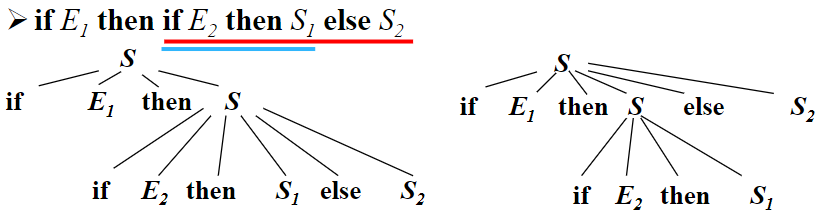文法二义性的消除方法：
不改变文法中原有的语法规则，仅加进一些语法的非形式规定。如运算符运算规定好优先顺序和结合规则构造一个等价的无二义性文法，把排除二义性的规则合并到原有文法中，改写原有的文法
【 PS：文法的二义性与语言的二义性是两个不同的概念 】
文法的二义性指存在某个句子是有二义性的语言的二义性指描述该语言的文法全部是二义性的，对它不存在无二义性的文法，这样的语言称为先天二义性的语言
文法和语言的分类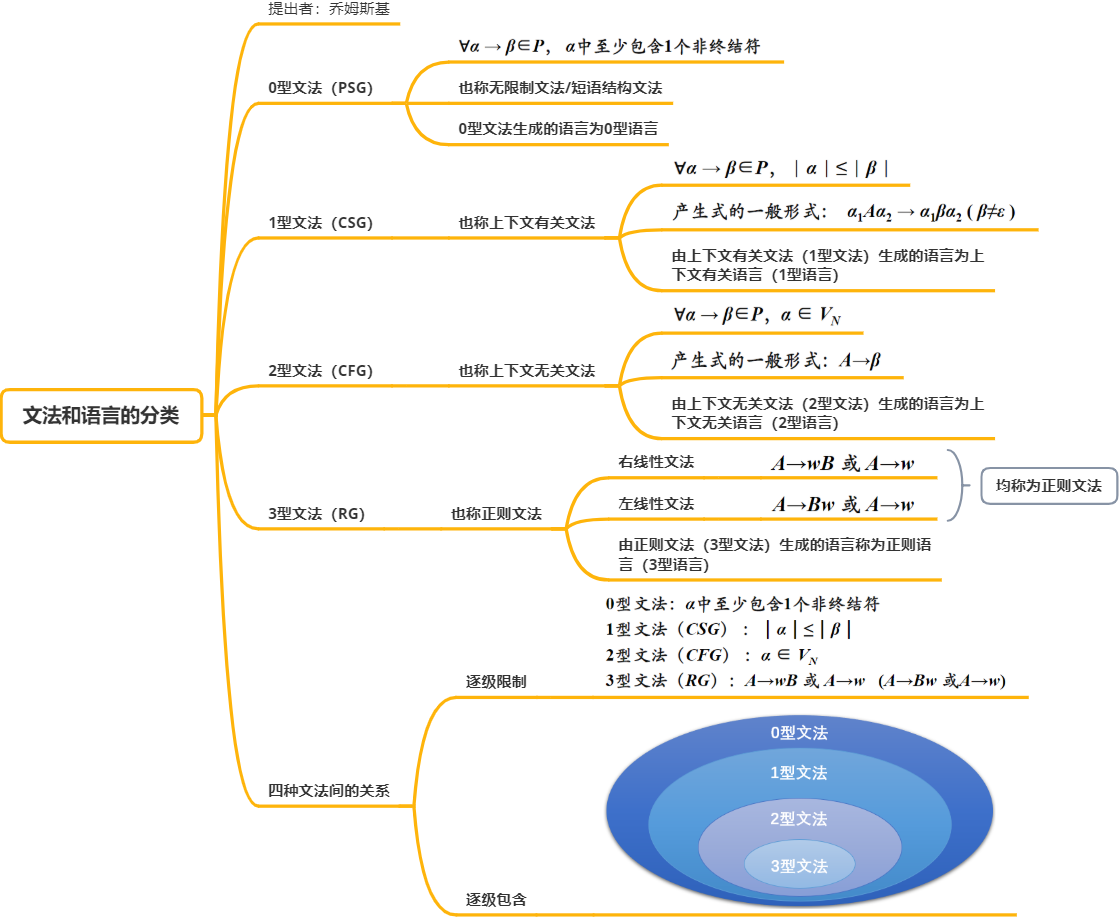常见题型
设计一个文法，定义一个已知的语言（已知语言，设计文法）：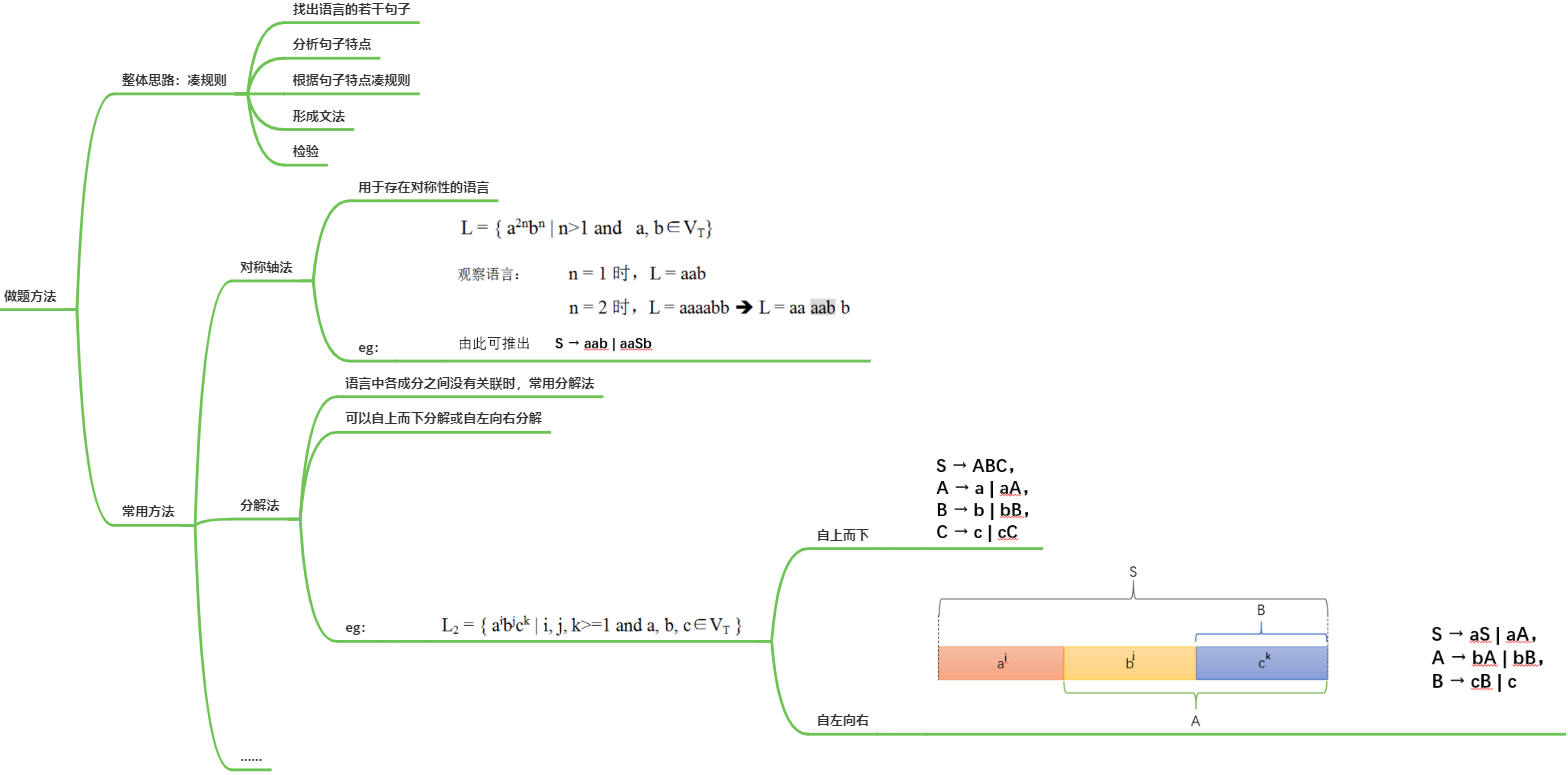已知一个文法，确定该文法所定义的语言（已知文法，确定语言）： 做题方法：从文法符号出发，反复连续地使用规则，对非终结符施行替换和展开，找出句子的规律，用式子或自然语言描述出来  求句型的短语、直接短语、句柄： 做题方法：画出语法分析树，通过剪枝，自左向右收集叶子结点，从而完成短语、直接短语、句柄的求解。eg：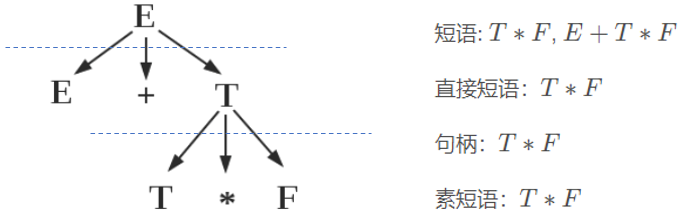判断文法的二义性： 做题方法：根据定义进行证明  文法的化简变换： 做题方法：根据限制条件进行化简

持续更新中…… 我是桐小白，一个摸爬滚打的计算机小白

展开全文语言 语法分析树 二义性
• 编译原理消除无用产生式的文法化简。用C++写的，实现消除无用产生式。
• 编译原理词法分析程序实验报告解析编译原理实验报告实验名称：编写词法分析程序实验类型：设计性实验指导教师：*****专业班级：软件工程1401姓 名：****学 号：**********实验地点：东六E座301实验成绩：___________...

编译原理词法分析程序实验报告解析
编译原理实验报告
实验名称：编写词法分析程序
实验类型：设计性实验
指导教师：*****
专业班级：软件工程1401
姓 名：****
学 号：**********
实验地点：东六E座301
实验成绩：_________________
日期： 2016 年 5 月 8 日
实验一
编写词法分析程序
一、实验目的
通过设计、调试词法分析程序，掌握词法分析程序的设计工具(有穷自动机)，进一步理解自动机理论
掌握正则文法和正则表达式转换成有穷自动机的方法及有穷自动机实现的方法
确定词法分析程序的输出形式及标识符与关键字的区分方法
加深对理论知识的理解
二、实验设计
设计原理：
对源程序代码从头到尾扫描，将符合词法语言规则的单词输出，包括：标识符、保留字、无符号整数、分界符、运算符、注释分离；判断程序的词法是否正确
TEST语言的词法规则如下：
1)、标识符：字母打头，后接任意字母或数字。
3)、无符号整数：由数字组成，但最高位不能为0，允许一位的0。
4)、分界符：(、)、;、{、}
5)、运算符：+、-、*、/、=、、>=、<=、!=、==
6)、注释符：/* */
设计方法：
用正则表达式或正则文法描述程序设计语言的词法规则，通常采用正则表达式；一个正则表达式对应一条词法规则
为每个正则表达式构造一个NFA，用来识别正则表达式描述的单词将每一个NFA合并、化简得到最简的DFA
将多个NFA合并为一个NFA
将NFA转换成等价的DFA。
最小化DFA
确定单词的输出形式。
化简后的DFA＋单词输出形式?构造词法分析程序
设计过程：
将TEST语言的六个语法规则分别转换成正则表达式
为每个正则表达式构造一个NFA，用来识别正则表达式描述的单词
将5个NFA转换成一个NFA，再将NFA化简确定化。
设计结果：
每一条TEST语言对应的正则表达式如下：
标识符：( a|b|……|z|A|B……|Z )( 0|1|……|9| a|b|……|z|A|B……|Z )*
保留字：标识符的子集
无符号整数： ( (1……|9 )( 0|1|……|9)* )|0
分界符：( | ) | ; | { | }
运算符：+ | - | * | / | = | < | > | >= | <= | != | ==
注释符：/*(其他)*/
NFA如图
化简、确定化的DFA
三、实验过程
将TEST语言的六个语法规则转换成正则表达式
将每个正则表达式装换成NFA,再将NFA合并化简
最终得到设计结果如上所示：
根据确定化的DFA编写代码
测试实验数据
三、实验结果
测试数据：
{
/*This a test program.*/
int abc;
int 123;
int A$@; int i; int n; int b,c; int 2a; int a2; read n; n = 012345; for (i=1;i<=n; i= i+1) { abc=abc+i; } if(i!=n) n = n+i; if (!n) b = b+c; /*The loop ended write abc; } 实验现象： 控制台显示的数据： 输出文本的数据： {{ intint IDabc ;; intint NUM123 ;; intint IDA Error$
Error@
;;
intint
IDi
;;
intint
IDn
;;
intint
IDb
Error,
IDc
;;
intint
NUM2
IDa
;;
intint
IDa2
;;
IDn
;;
IDn
==
NUM0
NUM12345
;;
forfor
((
IDi
==
NUM1
;;
IDi
<=<=
IDn
;;
IDi
==
IDi
++
NUM1
))
{{
IDabc
==
IDabc
++
IDi
;;
}}
ifif
((
IDi
!=!=
IDn
))
IDn
==
IDn
++
IDi
;;
ifif
((
Error!
IDn
))
IDb
==
IDb
++
IDc
;;
数据分析：
根据TEST语法规则，我们可以知道
int A$@;这一句  展开全文• 至少做到对下列已知的文法，用LL（1）分析法对任意输入的符号串进行分析： （1）E->TG （2）G->+TG （3）G->ε （4）T->FS （5）S->*FS （6）S->ε （7）F->(E) （8）F->I 高要求： 1、手动... LL(1)分析器 实验二 实验要求 简单要求： 至少做到对下列已知的文法，用LL（1）分析法对任意输入的符号串进行分析： （1）E->TG （2）G->+TG （3）G->ε （4）T->FS （5）S->*FS （6）S->ε （7）F->(E) （8）F->I 高要求： 1、手动输入文法 2、显示文法的非终结符的Follow集 3、显示规则的可选集合 3、构造预测分析表 4、对任意输入的符号串进行分析 记号和规定 空串：$符号栈终止符：#规定第一个产生式的左边那个非终结符就是开始符号
简单要求的固定文法（无左递归）
（1）E->TG
（2）G->+TG
（3）G->ε
（4）T->FS
（5）S->*FS
（6）S->ε
（7）F->(E)
（8）F->I
非终结符First集Follow集Select集E( I# )( IT( I+ # )( IG+ ε# )+ # )F( I+ * # )( IS* ε+ # )* + # )
产生式对应的SELECT集
非终结符产生式Slect集EE->TG( ITT->FS( IGG->+TG+GG->ε# )SS->*FS*SS->ε+ # )FF->(E)(FF->II
分析表
（I+*）#ETGTGsynchsynchTFSFSsynchsynchsynchG+TGεεF(E)IsynchsynchsynchsynchSε*FSεε
简单要求(固定文法递归的预测分析)
在主函数中构造一个RecursionSimple对象调用run方法即可运行
运行示例
[递归式固定文法]请输入需要分析的字符串：
I
[递归式固定文法]*****输入的字符串符合该文法*****success*****
[递归式固定文法]请输入需要分析的字符串：
***
[递归式固定文法]#####输入的字符串符不合该文法#####error#####
[递归式固定文法]请输入需要分析的字符串：
(I)
[递归式固定文法]*****输入的字符串符合该文法*****success*****

头文件 RecursionSimple.h
#pragma once

#include<iostream>
using namespace std;

//简单的递归版本 固定文法
class RecursionSimple{
public:
static const string name;
static enum over{Run,Error,Success};
int isOver;
char cur;
int len;
int pos;//当前指向的字符位置
string text;//需要分析的文本

//文法函数
void E();
void T();
void G();
void S();
void F();

//执行函数
void run();
//读入数据 在末尾插入#
void cinData();
//输入的字符串符合文法
void success();
//输入的内容不符合文法处理函数
void error();
//获取下一个字符 如果已经到了末尾则执行 error()
void next();
//输出结果
void coutResult();

};


源文件 RecursionSimple.cpp
#include "RecursionSimple.h"

#define IS_OVER if(isOver!=Run)return;

const string RecursionSimple::name = "[递归式固定文法]";

void RecursionSimple::E(){
IS_OVER
T();
G();
}

void RecursionSimple::T(){
IS_OVER
F();
S();
}

void RecursionSimple::G(){
IS_OVER
if(cur == '+'){
next();
T();
G();
} else if(cur != '+' && cur != ')' && cur != '#')error();
}

void RecursionSimple::S(){
IS_OVER
if(cur == '*'){
next();
F();
S();
} else if(cur != '+' && cur != ')' && cur != '#')error();
}

void RecursionSimple::F(){
IS_OVER
if(cur == '('){
next();
E();
if(cur == ')')next(); else error();

} else if(cur == 'I'){
next();
} else error();
}

void RecursionSimple::run(){
cout << "文法：\n" << "E->TG\n" << "G->+TG\n" << "G->ε\n" << "T->FS\n" << "S->*FS\n" << "S->ε\n" << "F->(E)\n" << "F->I\n";
cinData();//读入数据 并初始化
next();//获取第一个字符
E();//分析开始（首个非终结符函数）
if(cur == '#')success();
else error();
coutResult();//输出结果
}

void RecursionSimple::cinData(){
cout << name << "请输入需要分析的字符串：" << endl;
cin >> text;
text += '#';
pos = 0;
isOver = false;
len = text.length();
}

void RecursionSimple::success(){
isOver = Success;
}

void RecursionSimple::error(){
isOver = Error;
}

void RecursionSimple::next(){
if(pos < len){
cur = text[pos++];
return;
}
error();
}

void RecursionSimple::coutResult(){
if(isOver==Success)	cout << name << "*****输入的字符串符合该文法*****success*****" << endl;
else cout << name << "#####输入的字符串符不合该文法#####error#####" << endl;
}


高要求(任意文法非递归的预测分析)
读入文法确定终结符与非终结符去除左递归First集 Follow集判断是否为LL(1)文法(First与Follow元素不相交)Select集预测分析表分析
解析
读入文法: A -> @B a | c | $先输入左边 (在这里输入含有 # 的会结束文法输入)再输入右边 (同时输入各种可能 用 | 隔开 非终结符要在前面加@ ,#号结束本次右边输入)不可以包含下划线包含$的将自动转换为$单个字符 确定终结符与非终结符 去除左递归 先将间接左递归转换为直接左递归将所有直接左递归消除删除所有不可达的产生式 First集 Follow集 First集： 遍历每一个左部为x的产生式如果产生式右部第一个字符为终结符，则将其计入左部非终结符的First集如果产生式右部第一个字符为非终结符求该非终结符的First集将该非终结符的去掉$的First集计入左部的First集若存在$，继续往后遍历右部若不存在$，则停止遍历该产生式，进入下一个产生式若已经到达产生式的最右部的非终结符（即右部的First集都含有空串），则将$加入左部的First集处理数组中重复的First集中的终结符 Follow集： 如果x为起始符，x的Follow集添加#遍历每一个右部包含非终结符x的产生式如果x的下一个字符是终结符，添加进x的Follow集如果x的下一个字符是非终结符，把该字符的First集加入x的Follow集（不能加入空串）如果下一字符的First集有空串并且该产生式的左部不是x，则把左部的Follow集加入x的Follow集如果x已经是产生式的末尾，则把左部的Follow集添加到x的Follow集里 判断是否为LL(1)文法：First与Follow元素不相交 Select集： First集(除去ε) [+ Follow集(如果First集存在ε)] 预测分析表 遍历每一个左部为x的产生式如果x右边首字符串为 空串 则添加x的Follow集如果x右边首字符串为 终结符 则添加该终结符如果x右边首字符串为 非终结符 则添加x的First集 分析 分析栈放入 S# (S为起始非终结符) 文本栈放入 文本#获取分析栈顶l与文本栈顶ml如果为空串则直接出栈ll如果为终结符 看l是否等于m 相等则匹配（一起出栈） 否则报错l如果为非终结符 看l的分析表是否有m 有则一起出栈 否则报错分析栈与文本栈都全部出栈 则分析成功！ 运行示例 示例一 固定文法 [郭坤 软工一班 20192758] 欢迎使用LL1文法分析器，输入1使用固定文法，否则自定义文法:1 *****固定文法***** E->TG G->+TG|$
T->FS
S->*FS|$F->(E)|I First集 E:{ ( I } F:{ ( I } G:{$ + }
S:{ $* } T:{ ( I } Follow集 E:{ # ) } F:{ # ) * + } G:{ # ) } S:{ # ) + } T:{ # ) + } 是否为LL1文法：是 Select集 E:{ ( I } F:{ ( I } G:{ # ) + } S:{ # ) * + } T:{ ( I } 预测分析表 # ( ) * + I E @empty TG @empty @empty @empty TG F @empty (E) @empty @empty @empty I G$    @empty         $@empty +TG @empty S$    @empty         $*FS$    @empty
T    @empty        FS    @empty    @empty    @empty        FS

请输入待识别的符号串(空格分割，直到遇到#截止)
( I ) #

分析栈                    剩余输入串              推导式
E#                          (I)#               E->TG
TG#                          (I)#               T->FS
FSG#                          (I)#              F->(E)
(E)SG#                          (I)#              ( 匹配
E)SG#                           I)#               E->TG
TG)SG#                           I)#               T->FS
FSG)SG#                           I)#                F->I
ISG)SG#                           I)#              I 匹配
SG)SG#                            )#          S 空串出栈
G)SG#                            )#          G 空串出栈
)SG#                            )#              ) 匹配
SG#                             #          S 空串出栈
G#                             #          G 空串出栈
#                             #            @Accept!

示例二 任意文法
[郭坤 软工一班 20192758]
欢迎使用LL1文法分析器，输入1使用固定文法，否则自定义文法:0
输入文法(A -> a | @Bc [格式])[@B右边的非终结符前加@,输入#结束]
左边的非终结符:A @B c | d # B @A | f # #
右边的产生式 可以用 | 分割(每个终结符、非终结符、和'|' 都需要有空白分割！非终结符前加@):
*****结束右边的输入*****
A->Bc|d
输入文法(A -> a | @Bc [格式])[@B右边的非终结符前加@,输入#结束]
左边的非终结符:右边的产生式 可以用 | 分割(每个终结符、非终结符、和'|' 都需要有空白分割！非终结符前加@):
*****结束右边的输入*****
B->A|f
输入文法(A -> a | @Bc [格式])[@B右边的非终结符前加@,输入#结束]
左边的非终结符:*****结束文法输入*****
*****输入的文法如下*****
A->Bc|d
B->A|f

*****消除间接左递归*****
A->d|Ac|fc
B->A|f

*****消除直接左递归*****
A->dA_|fcA_
B->A|f
A_->$|cA_ *****删除不可达的文法***** A->dA_|fcA_ A_->$|cA_

First集
A:{ d f }
A_:{ $c } Follow集 A:{ # } A_:{ # } 是否为LL1文法：是 Select集 A:{ d f } A_:{ # c } 预测分析表 # B c d f A @empty @empty @empty dA_ fcA_ A_$    @empty       cA_    @empty    @empty

请输入待识别的符号串(空格分割，直到遇到#截止)
f c c c #

分析栈                    剩余输入串              推导式
A#                         fccc#            A_->fcA_
fcA_#                         fccc#              f 匹配
cA_#                          ccc#              c 匹配
A_#                           cc#             A_->cA_
cA_#                           cc#              c 匹配
A_#                            c#             A_->cA_
cA_#                            c#              c 匹配
A_#                             #         A_ 空串出栈
#                             #            @Accept!

代码
类头文件 ArbitraryGrammar.h
#pragma once
#include<iostream>
#include<vector>
#include<list>
#include<set>
#include<map>
#include<algorithm>
#include<iomanip>
#include<string>
using namespace std;

/*
- 空串：$- 符号栈终止符：# */ //产生式 struct Production{ string left; //产生式左边 非终结符 vector<vector<string>> right; //产生式右边 Production(string l,vector<vector<string>>r){ left = l;right = r; } Production(string l){ left = l; } string getRightString(int i){//获得右边所有元素联合的串 string s = ""; for(auto r : right[i])s += r; return s; } void insert(vector<string> temp){ right.push_back(temp); } void insert(string temp){ vector<string>v; v.push_back(temp); right.push_back(v); } string toString(){ string str = left + "->"; for(auto s : right)str += s; int len = right.size(); for(int i = 1;i < len;i++){ str += "|"; for(auto s : right[i])str += s; } return str; } }; //任意文法的LL(1)分析类 class ArbitraryGrammar{ public: ArbitraryGrammar(); //构造函数 void run(); //运行程序 void displayGrammarHint(string s); //打印系统提示语句 中间夹杂当前文法 void displayGrammar(); //打印当前的文法 void displayResultTable(); //打印分析结果表 void displayAnalyzedTable();//打印预测分析表 bool isNonTer(string x); //判断是否是 非终结符 bool isTer(string x); //判断是否是 终结符 void inputText(); //待分析的字符串输入 输入#号结束 void display(); //打印First集,Follow集,Select集 protected: int getNonTerPos(string x);//获取非终结符下标 int getTerPos(string x); //获取终结符下标 void init(); //初始化 void load(); //加载数据 调用其他方法 void inputGrammar(); //输入文法 输出#号结束 bool isLL1Grammar; //是否为LL1文法 bool isLoad; //是否加载了数据 bool isAnalyzed; //是否已经分析了输入的字符串 string Start; //起始符 //文本是否已经分析完毕 vector<Production> prod; //产生式集合 vector<string>text; //拆分后的 待分析文本 vector<vector<string>>resultTable;//分析后生成的结果分析表 0 分析栈 1 剩余输出串 2 推导式 vector<bool>used; //dfs看看哪些文法是访问了的 vector<set<string>> first; //First集 vector<set<string>> follow; //Follow集 vector<set<string>> select; //select集 map<string,int> terAndEnd; //终结符与# map<string,int> ter; //终结符 map<string,int> nonTer; //非终结符 string为非终结符 int为对应编号 vector<vector<int>> table; //分析表[非终结符][终结符]-> 存储的是产生式prod的下标 void judgeLL1Grammar(); //判断文法是否为LL(1)文法 First与Follow不相交 void getFirst(string x); //获取某个非终结符的First集 void getAllFirst(); //获取所有非终结符的First集 void getFollow(string x); //获取某个非终结符的Follow集 void getAllFollow(); //获取所有非终结符的Follow集 void getAllSelect(); //获取产生式的Select集 void getAnalyzTable(); //获取预测分析表 string getTableE(int prodPos,int rightPos); //获取预测分析表中的元素 -1 empty -2 synch void getResultTable(); //获取分析后的结果表 string getVectorString(vector<string> v);//将字符串集合整合为一个字符串 string getListString(list<string> li);//将字符串集合整合为一个字符串 void dfs(int x); void simplify(); void removeDirectly(int i); void removeIndirect(int i,int len);//消除间接做递归 产生式下标i 产生式数len void removeAllDirectly(); void removeAllIndirect(); void removeLeftRecursion(); //消除左递归 void resize(int len); //更新容器大小 };  类源文件 ArbitraryGrammar.cpp #include "ArbitraryGrammar.h" ArbitraryGrammar::ArbitraryGrammar(){ //init(); } bool ArbitraryGrammar::isNonTer(string x){ return nonTer.find(x) != nonTer.end(); } bool ArbitraryGrammar::isTer(string x){ return terAndEnd.find(x)!=terAndEnd.end(); } int ArbitraryGrammar::getNonTerPos(string x){ return nonTer.at(x); } int ArbitraryGrammar::getTerPos(string x){ return terAndEnd.at(x); } void ArbitraryGrammar::init(){ /*产生式 （1）E->TG （2）G->+TG （3）G->ε （4）T->FS （5）S->*FS （6）S->ε （7）F->(E) （8）F->I */ for(auto t : ter)terAndEnd.insert(t); int ii = terAndEnd.size(); terAndEnd["#"] = ii; Start = prod.left; int size = nonTer.size(); resize(size); isLoad = false; } void ArbitraryGrammar::inputText(){ //待分析的字符串输入 输入#号结束 cout << endl; cout << "请输入待识别的符号串(空格分割，直到遇到#截止)" << endl; vector<string>().swap(text); string sin;cin >> sin; while(sin.find('#') == string::npos){ text.push_back(sin); cin >> sin; } isAnalyzed = false;//输入了新的文本 设置为没有被分析 } void ArbitraryGrammar::inputGrammar(){ //输入所有非终结符 string s,ss; set<string>st; vector<Production>P; while(1){ cout << "输入文法(A -> a | @Bc [格式])[@B右边的非终结符前加@,输入#结束]" << endl; cout << "左边的非终结符:"; cin >> s; if(s.find('#') != string::npos){ cout << "*****结束文法输入*****" << endl; break; } if(s.find('@') != string::npos || s.find('_') != string::npos || s.find('$') != string::npos){
cout << "*****输入不合法 不能含有 @ 下划线 $*****" << endl; continue; } int pos; if(isNonTer(s)){ pos = getNonTerPos(s); } else{ int pos = nonTer.size(); nonTer[s] = pos; } st.erase(s);//消除未使用的非终结符 cout << "右边的产生式 可以用 | 分割(每个终结符、非终结符、和'|' 都需要有空白分割！非终结符前加@):" << endl; vector<vector<string>>V; vector<string>v; bool flag = 0; while(1){ cin >> ss; if(ss.find('#') != string::npos){ if(v.empty()){ cout << "*****产生式为空,不计入*****" << endl; flag = 2; break; } cout << "*****结束右边的输入*****" << endl; V.push_back(v); v.clear(); break; } if(ss.find('_') != string::npos){ cout << "*****输入不合法 不能含有下划线*****" << endl; flag = 1; break; } if(ss.find('$') != string::npos){
v.push_back("$"); continue; } if(ss.find('@') != string::npos){ if(ss != '@'){ cout << "*****输入不合法 @不在字符串首*****" << endl; flag = 1; break; } if(ss.size() == 1){ cout << "*****输入不合法 @后面没有非终结符串*****" << endl; flag = 1; break; } for(int i=1;i<ss.size();i++) if(ss[i] == '@'){ cout << "*****输入不合法 一个非终结符存在多个@ *****" << endl; flag = 1; break; } string nt = ss.substr(1); if(!isNonTer(nt))st.insert(nt);//计入非终结符序列 v.push_back(nt); continue; } if(ss == "|" && !v.empty()){ V.push_back(v); v.clear(); continue; } v.push_back(ss); } if(flag)continue; //成功输入一组文法 Production pr = Production(s,V); for(auto vv : V){ for(auto x : vv){ if(!isNonTer(x) && x != "$"){
int ii = ter.size();
ter[x] = ii;
}
}
}
P.push_back(pr);
cout << pr.toString() << endl;
}

displayGrammarHint("输入的文法如下");

if(!st.empty()){
cout << "存在终结符没有对应的产生式！--> ";
for(auto x : st)cout << x << " ";
cout << endl << "请重新输入文法！" << endl;
nonTer.clear();
ter.clear();
prod.clear();
inputGrammar();
}

//更新prod
prod.swap(P);

}

void ArbitraryGrammar::run(){

cout << "[郭坤 软工一班 20192758]" << endl;
cout << "欢迎使用LL1文法分析器，输入1使用固定文法，否则自定义文法:";
string sin;
getline(cin,sin);
if(sin == "1"){
prod.push_back(Production("E",{{"T","G"}}));
prod.push_back(Production("G",{{"+","T","G"},{"$"}})); prod.push_back(Production("T",{{"F","S"}})); prod.push_back(Production("S",{{"*","F","S"},{"$"}}));
prod.push_back(Production("F",{{"(","E",")"},{"I"}}));

ter["+"] = 0;
ter["*"] = 1;
ter["("] = 2;
ter[")"] = 3;
ter["I"] = 4;

nonTer["E"] = 0;
nonTer["G"] = 1;
nonTer["T"] = 2;
nonTer["S"] = 3;
nonTer["F"] = 4;

init();
displayGrammarHint("固定文法");

} else{
inputGrammar();
init();
removeLeftRecursion();
}

}

getAllFirst();
getAllFollow();

judgeLL1Grammar();

getAllSelect();

display();

if(isLL1Grammar){
getAnalyzTable();
displayAnalyzedTable();

while(true){
inputText();
getResultTable();
displayResultTable();
cout << endl << "是否继续分析一组文本? y|Y" << endl;
string s;
cin >> s;
if(s != 'y' && s != 'Y')break;

}

}
}

void ArbitraryGrammar::display(){
cout << endl;
cout << "First集" << endl;
for(auto x : nonTer){
cout << x.first << ":{ ";
for(auto f : first[x.second]){
cout << f << " ";
}
cout << "}" << endl;
}

cout << endl;
cout << "Follow集" << endl;
for(auto x : nonTer){
cout << x.first << ":{ ";
for(auto f : follow[x.second]){
cout << f << " ";
}
cout << "}" << endl;
}

cout << endl;
cout << "是否为LL1文法：" << ( isLL1Grammar ? "是" : "不是" ) << endl;

cout << endl;
cout << "Select集" << endl;
for(auto x : nonTer){
cout << x.first << ":{ ";
for(auto f : select[x.second]){
cout << f << " ";
}
cout << "}" << endl;
}
}

}

void ArbitraryGrammar::displayResultTable(){
if(isLL1Grammar){

if(!isAnalyzed)getResultTable();//如果没有分析 则进行分析

//分析表结果表输出
int w0 = 20,w1 = 30,w2 = 20;
cout << endl;
cout << setw(w0) << "分析栈" << setw(w1) << "剩余输入串" << setw(w2) << "推导式" << endl;
for(int i = 0;i < resultTable.size();i++){
cout << setw(w0) << resultTable[i] << setw(w1) << resultTable[i] << setw(w2) << resultTable[i] << endl;
}
} else{
cout << "当前文法不合法LL(1)规范，不可以打印LL(1)结果分析表" << endl;
}
}

void ArbitraryGrammar::displayGrammar(){
for(auto & p : prod)cout << p.toString() << endl;
}

void ArbitraryGrammar::displayGrammarHint(string s){
cout << "*****" << s << "*****" << endl;
displayGrammar();
cout << endl;
}

void ArbitraryGrammar::displayAnalyzedTable(){
if(isLL1Grammar){
int width = 10;
cout << endl;
cout << "预测分析表" << endl;

//打印终结符
cout << setw(width) << "";
for(auto s : terAndEnd){
cout << setw(width) << s.first;
}

cout << endl;
//打印预测的产生式
for(auto nt : nonTer){
cout << setw(width) << nt.first;
int pos = getNonTerPos(nt.first);
for(auto t : terAndEnd){
int i = table[nt.second][t.second];
cout << setw(width) << getTableE(pos,i);
}
cout << endl;
}
}
}

void ArbitraryGrammar::judgeLL1Grammar(){
//First与Follow都不相交的才属于LL1文法
int len = nonTer.size();
for(int i = 0;i < len;i++){
set<string>tmp;
set_intersection(first[i].begin(),first[i].end(),follow[i].begin(),follow[i].end(),inserter(tmp,tmp.begin()));
if(!tmp.empty()){
isLL1Grammar = false;
return;
}
}
isLL1Grammar = true;
}

void ArbitraryGrammar::getFirst(string x){
bool flag = 0;  //记录非终结符的First集是否有空串
int tot = 0;    //记录一个非终结符产生式含有空串的产生式
int pos = getNonTerPos(x);//该非终结符对应的下标

for(auto v : prod[pos].right){
//如果右部的第一个字符是终结符
if(isTer(v)){
first[pos].insert(v);
}
//如果是非终结符
else{
//从左到右遍历右部
for(int j = 0;j < v.size();j++){
//如果遇到终结符，直接将该终结符放入first集 并结束
if(isTer(v[j])){
first[pos].insert(v[j]);
break;
}
//遇到空串
if(v[j] == '$'){ //是最后一个符号 则加入first中 if(j == v.size() - 1){ first[pos].insert("$");
break;
}
//不是最后一个符号 ---其实可以去除它！
continue;
}
//不是终结符，求该非终结符的First集
getFirst(v[j]);
for(string it : first[getNonTerPos(v[j])]){
if(it == '$'){//查看是否有空串在该非终结符的first中 flag = 1; } else{//将该非终结符的first的非空串元素添加到first集中 first[pos].insert(it); } } //没有空串就不必再找下去了 if(flag == 0)break; else{//有空串 则继续循环 flag = 0;//更新状态 tot++; } } //如果右部所有符号的First集都有空串] = 则符号x的First集也有空串 if(tot == v.size()){ first[pos].insert("$");
}
}
}

}

void ArbitraryGrammar::getAllFirst(){
//getFirst
for(auto i : nonTer)getFirst(i.first);
}

void ArbitraryGrammar::getAllFollow(){
//起始非终结符的follow放入#
follow.insert("#");
//getFollow
for(auto i : nonTer)getFollow(i.first);
}

void ArbitraryGrammar::getFollow(string x){
int pos = getNonTerPos(x);

//找到非终结符x出现的位置
for(int i = 0;i < prod.size();i++){
for(auto v : prod[i].right){
int index = -1;
int len = v.size();
for(int j = 0;j < len;j++){
if(v[j] == x){
index = j;
break;
}
}
if(index == -1)continue;//没有包含x结束本次循环
//如果找到了x] = 并且它不是最后一个字符
if(index < len - 1){
//如果下一个字符是终结符，添加进x的Follow集
string next = v[index + 1];
if(isTer(next))follow[pos].insert(next);
else{
//如果下一个字符是非终结符
bool flag = 0;
//遍历下一个字符的First集
for(string it : first[getNonTerPos(next)]){
if(it == '$')flag = 1; else follow[pos].insert(it); } //如果有空串并且左部不是它本身(防止陷入死循环)] = 当前非终结符的Follow集是x的Follow集 string l = prod[i].left; if(flag && l != x){ getFollow(l); for(string it : follow[getNonTerPos(l)]){ follow[pos].insert(it); } } } } else if(index == len - 1 && x != prod[i].left){ //如果x在产生式的末尾，则产生式左部的Follow集应该添加到x的Follow集里 string l = prod[i].left; getFollow(l); for(string it : follow[getNonTerPos(l)]){ follow[pos].insert(it); } } } } } void ArbitraryGrammar::getAllSelect(){ //Slect集 = First集(除去ε) [+ Follow集(如果First集存在ε)] for(auto m : nonTer){ string x = m.first; int pos = m.second; if(first[pos].find("$") != first[pos].end()){
//First存在空串
set_union(first[pos].begin(),first[pos].end(),follow[pos].begin(),follow[pos].end(),inserter(select[pos],select[pos].begin()));
//取出select中的空串 $select[pos].erase("$");
} else{//不存在空串
for(auto s : first[pos])select[pos].insert(s);
}
}
}

void ArbitraryGrammar::getAnalyzTable(){

for(int i = 0;i < prod.size();i++){
for(int j = 0;j < prod[i].right.size();j++){
string tmp = prod[i].right[j];
int pos = getNonTerPos(prod[i].left);
//如果产生式右部的第一个字符串是终结符
if(isTer(tmp) || tmp == '$'){ //该终结符是空串，遍历左部的follow集，更新table if(tmp == '$'){
for(auto f : follow[pos])table[pos][getTerPos(f)] = j;
} else{//该终结符不是空串，更新table
table[pos][getTerPos(tmp)] = j;
}
} else{
//如果产生式右部的第一个字符是非终结符，遍历它的First集，更新table
int tmpPos = getNonTerPos(tmp);
for(auto f : first[tmpPos]){//添加 除空串$以外的所有元素 if(f != '$')table[pos][getTerPos(f)] = j;
}
//如果有空串，遍历左部的follow集，更新table
if(first[tmpPos].find("$") != first[tmpPos].end()){ for(auto f : follow[pos])table[pos][getTerPos(f)] = j; } } } } } string ArbitraryGrammar::getTableE(int prodPos,int rightPos){ if(rightPos == -1)return "@empty"; //if(1 == -2)return "@synch"; return prod[prodPos].getRightString(rightPos); } void ArbitraryGrammar::getResultTable(){ if(isAnalyzed)return;//分析过就不需要再分析了 isAnalyzed = true; auto rs = &resultTable; //重置结果表 vector<vector<string>>().swap(*rs); rs->resize(3); list<string>a,b;//a-->分析栈 b->剩余输出串栈 auto A = &rs->at(0),B = &rs->at(1),C = &rs->at(2); //把开始符和#push进分析栈 a.push_back(Start); a.push_back("#"); //把整个串push进剩余符号vector for(auto i : text)b.push_back(i); b.push_back("#"); //如果剩余输入串长度不为0，就一直循环 while(b.size() > 0){ //输出分析栈内容 A->push_back(getListString(a)); //输出剩余输入串内容 B->push_back(getListString(b)); //获取分析栈首元素与剩余输入串首元素 string sa = a.front(),sb = b.front(); int pa; if(sa == sb){ if(sa == "#"){//如果可以匹配，并且都为# C->push_back("@Accept!");//输入串符合文法！ return; } else{//如果可以匹配，并且都为终结符 a.pop_front(); b.pop_front(); C->push_back(sb + " 匹配"); } }else if(isNonTer(sa)&& isTer(sb) &&table[pa = getNonTerPos(sa)][getTerPos(sb)] > -1){ //如果在预测分析表中有值 int index = table[pa][getTerPos(sb)]; a.pop_front(); if(getTableE(pa,index) != "$"){
//E#->TG# 倒叙插入
for(int i = prod[pa].right[index].size() - 1;i >= 0;i--){
a.push_front(prod[pa].right[index][i]);
}
C->push_back(prod[pa].left + "->" + getVectorString(prod[pa].right[index]));
} else{//空串出栈
C->push_back(sa + " 空串出栈");
}
} else{
C->push_back("@Error!");
return;
}
}
}

string ArbitraryGrammar::getVectorString(vector<string> v){
string tmp = "";
for(auto i : v)tmp += i;
return tmp;
}

string ArbitraryGrammar::getListString(list<string> li){
string tmp = "";
for(auto i : li)tmp += i;
return tmp;
}

//消除间接左递归
void ArbitraryGrammar::removeIndirect(int pa,int len){

for(int pb = pa; pb < len; pb++){
//相互循环遍历 一层层的消除间接左递归
bool flag = false;
vector<vector<string>> tmp;
vector<vector<string>> & Ar = prod[pa].right;//检查的产生式右边Ar
vector<vector<string>> & Br = prod[pb].right;//搜寻的产生式右边Br
string Bl = prod[pb].left;//搜寻的产生式 左边
//A->Bc B->Cd => A->Cdc
for(auto & a : Ar)
if(a == Bl){//Ar中发现有以Bl开头的式子
//将B中非终结符式子Cd筛选出来
for(auto & b : Br)
if(b!=Bl&&b!="$") tmp.push_back(b); //找到Bl开头元素 flag = true; break; } if(flag){ //使用n--反向读取方式 避免因为删除导致的数组下标错误 int n = Ar.size(); while(n--){ if(Ar[n] == Bl){//Ar中发现有以Bl开头的式子 //取出tmp中的元素Cd加上a中的c 然后放入Ar中 Cdc for(auto t : tmp){ int nn = Ar[n].size(); for(int k = 1;k < nn;k++) t.push_back(Ar[n][k]); //放入Ar中 A->CDc Ar.push_back(t); } //删除A->Bc项 Ar.erase(Ar.begin() + n); } } } } } void ArbitraryGrammar::removeAllDirectly(){ //固定了原本产生式数量 即使后续增加不会改变 因此之后遍历原有的产生式 int len = prod.size(); for(int i = 0;i < len;i++)removeDirectly(i); //扩容 len = prod.size();//获取扩容后的大小 resize(len); displayGrammarHint("消除直接左递归"); } void ArbitraryGrammar::removeAllIndirect(){ int len = prod.size(); for(int i = 0;i < len;i++)removeIndirect(i,len); displayGrammarHint("消除间接左递归"); } void ArbitraryGrammar::removeLeftRecursion(){ //先消除所有间接左递归 再消除所有 直接左递归 removeAllIndirect(); removeAllDirectly(); simplify(); } void ArbitraryGrammar::resize(int len){ first.resize(len); follow.resize(len); select.resize(len); table.resize(len); for(auto & i : table){ i.resize(terAndEnd.size()); for(auto & x : i)x = -1;//设置为-1 empty } } //消除直接左递归 void ArbitraryGrammar::removeDirectly(int pos){ string l = prod[pos].left;//获取左边 //遍历看看有没有左递归元素 bool isLeftRecursion = false; for(auto & i : prod[pos].right){ if(i == l){ isLeftRecursion = true; break; } } if(!isLeftRecursion)return; //创建A_并放入prod中 string l_ = l + '_'; auto A_ = Production(l_); //nonTer 添加A_ nonTer[l_] = prod.size(); prod.push_back(A_); //----这里不知道为什么 不能放在前面声明r（会导致到下面循环时 r变空 只能放在这里了） auto & r_ = prod[prod.size() - 1].right; //添加空串到A_中 r_.push_back({"$"});
auto &r = prod[pos].right;

//遍历A右边 寻找递归左元素
for(int x = 0;x < r.size();x++){
if(r[x] == "\$")continue;//跳过空串
if(r[x] == l){//A->Ab => A_->bA_
vector<string>v;
for(int i = 1;i < r[x].size();i++)
v.push_back(r[x][i]);//b
v.push_back(l_);//bA_
r_.push_back(v);//添加A_->nA_
r.erase(r.begin()+x);//删掉A->Ab
x--;//回退一格
} else{//A->c => A->cA_
r[x].push_back(l_);//cA_
}
}
}

void ArbitraryGrammar::dfs(int x){
if(used[x]) return;
used[x] = 1;
for(vector<string> &v : prod[x].right)
for(string &s:v)
if(isNonTer(s))
dfs(getNonTerPos(s));
}

//化简 没有使用的文法扔掉
void ArbitraryGrammar::simplify(){
//还没做好
vector<bool>().swap(used);
used.resize(prod.size());

dfs(0);

vector<Production> res;
for(int i = 0; i < prod.size(); i++)
if(used[i])
res.push_back(prod[i]);

prod.swap(res);

resize(prod.size());

nonTer.clear();
for(int p = 0;p < prod.size();p++)
nonTer[prod[p].left] = p;

displayGrammarHint("删除不可达的文法");
}


主函数 main.cpp
#include <iostream>
#include <cmath>
#include "ArbitraryGrammar.h"
using namespace std;

int main(){

ArbitraryGrammar ag = ArbitraryGrammar();
ag.run();

}


展开全文经验分享 c++
• 一、编译原理——编译基础知识 相关概念： 编译 编译就是高级语言翻译成低级语言的过程，翻译完全部代码后执行。 解释 解释也是翻译的过程，但和编译不同，解释时逐句翻译，一边解释一边执行。 编译的过程 ...
• 编译原理LL1文法在上次介绍了词法分析之后，这次介绍如何将一个普通文法，消除左递归，提取左因子，从而得到LL1文法。先贴出最终的效果图 这里给出具体的实现要求1、将一个可转换非LL（1）文法转换为LL（1）文法，...list
• ## 文法化简与改造

热门讨论 2007-12-16 20:50:33
文法化简的程序，实现文法化简文法改造，的消除空产生式，消除单产生式，消除无用产生式的功能
• （DFA化简矩阵->最小DFA->）左线性文法。 例子： 给定右线性文法G[S]: S→ 0S | 1S | 1A | 0B A→ 1C | 1 B→ 0C | 0 C→ 0C | 1C | 0 | 1 求出一个与G[S]等价的左线性文法。 首先画出其FA； 然后是...
• 编译原理-医学信息工程专业一、 文法的直观概念1.2.二、符号和符号串三、文法和语言的形式定义四、文法的类型五、上下文无关文法及其语法数六、语句的分析七、有关文法实际应用的一些说明 一、 文法的直观概念 1. 2....
• 对于任意上下文无关的文法消除左递归 问题分析 一、产生式直接消除左递归 形如P→Pα|β可以通过直接消除转化为：  P→βP′P′→αP′|ϵ 二、产生式间接消除左递归 有时候虽然形式上产生...
• https://www.bilibili.com/video/BV1ft4y1X7p6?p=2 编译系统组成 产生下列语言的文法 最左推导最右推导，子树，直接子树 二义性文法 短语，直接短语，句柄，素短语 化简文法
• 这个编译原理课程设（LL1文法和语法分析器）是有图形界面的，界面很人性化，界面窗口上各个生成的过程都有，其中包括原文法、化简后的文法、预测分析表、分析过程都有LL1 语法分析器
• 一个文法G，若存在P经过一次或多次推导得到Pa（即能推导出以P开头的式子）， 则称G是左递归的。 　左递归分为直接左递归和间接左递归。 　直接左递归经过一次推导就可以看出文法存在左递归，如P→Pa｜b。 　...
• 对NFA的考量是困难的，对DFA的考量则是无比清晰的。...包含了之前的终止状态的新状态是新的终止状态，包含了之前的起始状态的新状态是起始状态，所以终止状态是B，起始状态是B，故化简后的DFA是：NFA 形式语言与自动机
• 文法G中的非终结符A，对某个文法符号序列α存在推导A=+>AαA=^+>AαA=+>Aα，则称G是左递归的。若G中有形如A→Aα的产生式，则称该产生式对A直接左递归 首先，整理A产生式为如下形式: A→ Aα1|Aα2|…|...
• 对于任意上下文无关的文法消除左递归 问题分析 一、产生式直接消除左递归 形如P→Pα|β可以通过直接消除转化为： P→βP′P′→αP′|ϵ 二、产生式间接消除左递归 有时候虽然形式上产生式没有递归，但是...
• 河北工业大学编译原理期末复习.docx考试题型填空 24简答 4*416解答 4*156Chapter 1 重要概念1.什么编译程序P3答编译程序的主要功能是把用高级语言编写的源程序翻译为等价的目标程序。2.编译程序的工作过程(6 个阶段)...
• 编译原理学习笔记, 超万字的编译原理知识总结...............
• ## 编译原理学习基本概念汇总

千次阅读 多人点赞 2015-07-22 14:04:10
对于计算机专业的学生来说，肯定听说过或者上过一门课，叫做——编译原理，被称为计算机专业的天书，反正不管是学习这门课的时候，还是现在，我都是没搞懂其中的技术和知识。但就期末考试而言，提前做了几道题目，...基本概念
• 1、参考书籍：《编译原理》第三版 清华大学出版社 1、本文内容全部来源于开源社区 GitHub和以上博主的贡献，本文也免费开源（可能会存在问题，评论区等待大佬们的指正） 2、本文目的：开源共享 抛砖引玉 一起学习 3...
• ## 编译原理期末复习

千次阅读 多人点赞 2019-01-12 10:17:26
• ## 编译原理

千次阅读 多人点赞 2019-06-05 23:00:32
编译原理基本知识总结，基于MOOC陈鄞老师的课程。
• 2.3.4 DFA的化简 2.4 从正规式到有限自动机 2.5 词法分析器的生成器 第3章 语法分析 3.1 上下文无关文法 3.1.1上下文无关文法的定义 3.1.2 推导 3.1.3 分析树 3.1.4 二义性 3.2 语言和文法 3.2.1 正规式和上......